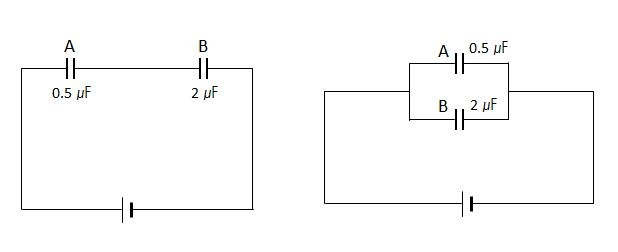# Capacity of CapacitorThe above two diagrams show capacitors A and B, with their respective capacities $0.5$ $\mu$F and $2$ $\mu$F, connected to an electric circuit in two different ways. The one on the left is a series connection, while the one on the right is a parallel connection. What are the combined capacitance in the above left($C_s$) and right($C_p$) diagrams, respectively?

×